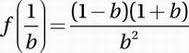# SAT Math Multiple Choice Question 711: Answer and Explanation

### Test Information

Question: 711

6. Let function f(x) be defined by the equation f(x) =x2 - 1. If b is a positive real number, then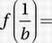• A.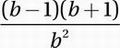• B.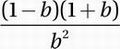• C.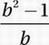• D.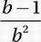Explanation:

B

f(x) = x2 - 1

Substitute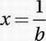: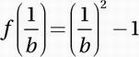Simplify: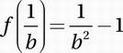Get common denominator: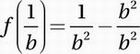Subtract fractions: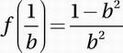Factor numerator: